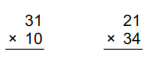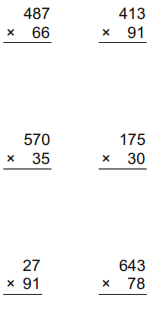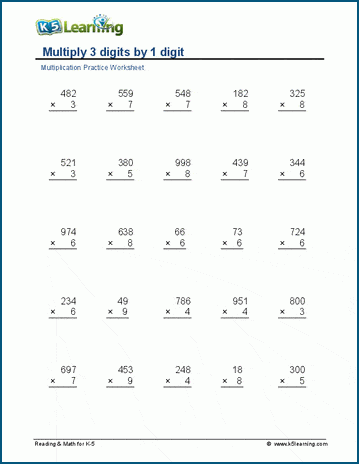# Multi-digit multiplication worksheets

## Multiplication and regrouping

These worksheets proceed stepwise from simple multiplying small numbers mentally to multiplication of large numbers in columns with regrouping.  Emphasis is on the regrouping algorithm.

We also have multiplication facts worksheets and thousands of math worksheets by grade level.

## Multiplication (no regrouping)

Multiply in columns (no regrouping)Horizontal multiplication (no regrouping)

## Multiply in columns

Multiply 2 x 1 digitsMultiply 3 x 1 digits
Multiply 4 x 1 digits
Multiply 2 x 2 digits
Multiply 3 x 2 digits
Multiply 3 x 3 digits
Multiply 4 x 2 digits
Multiply 4 x 3 digits
Multiply 4 x 4 digits
Multiply 5 or more digits

## Multiples of 10, 100, 1000

Multiplying by 10 10 x 278 =
Multiplying by 100 717 x 100 =
Multiplying by 1,000 587 x 1,000 =
Multiplying by multiples of 10 38 x 40 =
Multiplying by multiples of 100 57 x 400 =
Multiplying by multiples of 1000 98 x 4,000 =
Multiply whole tens  90 x 40 =
Multiply whole tens by whole hundreds and thousands 60 x 8,000 =
Multiply whole hundreds 400x700 =
Multiply whole thousands 5,000 x 9,000 =
Multiplying by whole tens and hundreds- missing factor ___ x 200 = 3,400Sample multiplication worksheet

What is K5?

K5 Learning offers free worksheets, flashcards and inexpensive workbooks for kids in kindergarten to grade 5. Become a member to access additional content and skip ads.